Permutation and Combinations (Part - 1)

# Permutation and Combinations (Part - 1) Notes | Study Business Mathematics and Logical Reasoning & Statistics - CA Foundation

## Document Description: Permutation and Combinations (Part - 1) for CA Foundation 2022 is part of Business Mathematics and Logical Reasoning & Statistics preparation. The notes and questions for Permutation and Combinations (Part - 1) have been prepared according to the CA Foundation exam syllabus. Information about Permutation and Combinations (Part - 1) covers topics like and Permutation and Combinations (Part - 1) Example, for CA Foundation 2022 Exam. Find important definitions, questions, notes, meanings, examples, exercises and tests below for Permutation and Combinations (Part - 1).

Introduction of Permutation and Combinations (Part - 1) in English is available as part of our Business Mathematics and Logical Reasoning & Statistics for CA Foundation & Permutation and Combinations (Part - 1) in Hindi for Business Mathematics and Logical Reasoning & Statistics course. Download more important topics related with notes, lectures and mock test series for CA Foundation Exam by signing up for free. CA Foundation: Permutation and Combinations (Part - 1) Notes | Study Business Mathematics and Logical Reasoning & Statistics - CA Foundation
 1 Crore+ students have signed up on EduRev. Have you?

LEARNING OBJECTIVES
After reading this Chapter a student will be able to understand —

• difference between permutation and combination for the purpose of arranging different objects;
• number of permutations and combinations when r objects are chosen out of n different objects.
• meaning and computational techniques of circular permutation and permutation with restrictions.

INTRODUCTION

In this chapter we will learn problem of arranging and grouping of certain things, taking particular number of things at a time. It should be noted that (a, b) and (b, a) are two different arrangements, but they represent the same group. In case of arrangements, the sequence or order of things is also taken into account.
The manager of a large bank has a difficult task of filling two important positions from a group of five equally qualified employees. Since none of them has had actual experience, he decides to allow each of them to work for one month in each of the positions before he makes the decision. How long can the bank operate before the positions are filled by permanent appointments?
Solution to above - cited situation requires an efficient counting of the possible ways in which the desired outcomes can be obtained. A listing of all possible outcomes may be desirable, but is likely to be very tedious and subject to errors of duplication or omission. We need to devise certain techniques which will help us to cope with such problems. The techniques of permutation and combination will help in tackling problems such as above.

FUNDAMENTAL PRINCIPLES OF COUNTING

(a) Multiplication Rule: If certain thing may be done in ‘m’ different ways and when it has been done, a second thing can be done in ‘n ‘ different ways then total number of ways of doing both things simultaneously = m × n.
Eg. if one can go to school by 5 different buses and then come back by 4 different buses then total number of ways of going to and coming back from school = 5 × 4 = 20.

(b) Addition Rule : It there are two alternative jobs which can be done in ‘m’ ways and in ‘n’ ways respectively then either of two jobs can be done in (m + n) ways.
Eg. if one wants to go school by bus where there are 5 buses or to by auto where there are 4 autos, then total number of ways of going school = 5 + 4 = 9.
Note :- 1)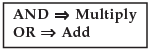2) The above fundamental principles may be generalised, wherever necessary.

Definition : The factorial n, written as n! or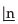, represents the product of all integers from 1 to n both inclusive. To make the notation meaningful, when n = o, we define o! or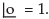Thus, n! = n (n – 1) (n – 2) ….. …3.2.1

Example 1 : Find 5! ; 4! and 6!

Solution : 5! = 5 × 4 × 3 × 2 × 1 = 120; 4! = 4 × 3 × 2 × 1 = 24; 6! = 6 × 5 × 4 × 3 × 2 × 1 = 720.

Example 2 : Find 9 ! / 6 ! ; 10 ! / 7 !.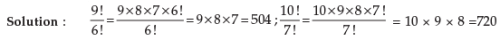Example 3 : Find x if 1/9 ! + 1/10 ! = x/11 !

Solution : 1/9! (1 + 1/10) = x/11 × 10 × 9! Or, 11/10 = x/11 × 10 i.e., x = 121

Example 4 : Find n if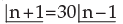Solution: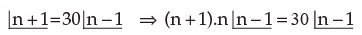or, n2 + n = 30 or, n2 + n – 30 or, n2 + 6n – 5n – 30 = 0      or,   (n + 6) (n – 5) = 0 either n = 5 or n = –6. (Not possible) ∴ n = 5.

PERMUTATIONS

A group of persons want themselves to be photographed. They approach the photographer and request him to take as many different photographs as possible with persons standing in different positions amongst themselves. The photographer wants to calculate how many films does he need to exhaust all possibilities? How can he calculate the number?
In the situations such as above, we can use permutations to find out the exact number of films.

Definition: The ways of arranging or selecting smaller or equal number of persons or objects from a group of persons or collection of objects with due regard being paid to the order of arrangement or selection, are called permutations.
Let us explain, how the idea of permutation will help the photographer. Suppose the group consists of Mr. Suresh, Mr. Ramesh and Mr. Mahesh. Then how many films does the photographer need? He has to arrange three persons amongst three places with due regard to order. Then the various possibilities are (Suresh, Mahesh, Ramesh), (Suresh, Ramesh, Mahesh), (Ramesh, Suresh, Mahesh), (Ramesh, Mahesh, Suresh), (Mahesh, Ramesh, Suresh) and (Mahesh, Suresh, Ramesh ). Thus there are six possibilities. Therefore he needs six films. Each one of these possibilities is called permutation of three persons taken at a time.

This may also be exhibited as follows :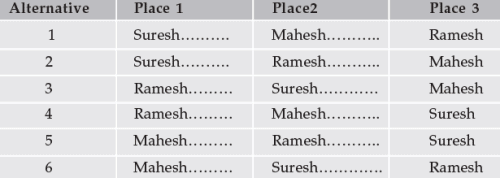with this example as a base, we can introduce a general formula to find the number of permutations.

Number of Permutations when r objects are chosen out of n different objects. (Denoted by nPr or nPr or P(n, r) ) :

Let us consider the problem of finding the number of ways in which the first r rankings are secured by n students in a class. As any one of the n students can secure the first rank, the number of ways in which the first rank is secured is n.

Now consider the second rank. There are (n – 1) students left, the second rank can be secured by any one of them. Thus the different possibilities are (n – 1) ways. Now, applying fundamental principle, we can see that the first two ranks can be secured in n (n – 1) ways by these n students.
After calculating for two ranks, we find that the third rank can be secured by any one of the remaining (n – 2) students. Thus, by applying the generalized fundamental principle, the first three ranks can be secured in n (n – 1) (n – 2) ways .

Continuing in this way we can visualise that the number of ways are reduced by one as the rank is increased by one. Therefore, again, by applying the generalised fundamental principle for r different rankings, we calculate the number of ways in which the first r ranks are secured by n students as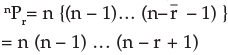Theorem: The number of permutations of n things chosen r at a time is given by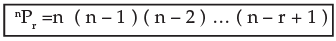where the product has exactly r factors.

RESULTS

1. Number of permutations of n different things taken all n things at a time is given by

nPn = n (n – 1) (n – 2) …. (n – n + 1)
=n (n – 1) (n – 2) ….. 2.1 = n!

2. nPr using factorial notation. nPr = n. (n – 1) (n – 2) ….. (n – r + 1)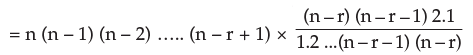= n!/( n – r )!
Thus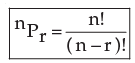3. Justification for 0! = 1. Now applying r = n in the formula for nPr, we get
nPn = n!/ (n – n)! = n!/0!
But from Result 1 we find that nPn = n!. Therefore, by applying this we derive, 0! = n! / n! = 1

Example 1 : Evaluate each of 5P3, 10P2, 11P5.

Solution : 5P3 = 5×4× (5–3+1) = 5 × 4 × 3 = 60,
10P2 = 10 × …. × (10–2+1) = 10 × 9 = 90,
11P= 11! / (11 – 5)! = 11 × 10 × 9 × 8 × 7 × 6! / 6! = 11 × 10 × 9 × 8 × 7 = 55440.

Example 2 : How many three letters words can be formed using the letters of the words (a) square and (b) hexagon?

(Any arrangement of letters is called a word even though it may or may not have any meaning or pronunciation).

Solution : (a) Since the word ‘square’ consists of 6 different letters, the number of permutations of choosing 3 letters out of six equals 6P3 = 6 × 5 × 4 = 120.

(b) Since the word ‘hexagon’ contains 7 different letters, the number of  permutations is 7P3 = 7 × 6 × 5 = 210.

Example 3 : In how many different ways can five persons stand in a line for a group photograph?

Solution : Here we know that the order is important. Hence, this is the number of permutations of five things taken all at a time. Therefore, this equals
5P5 = 5! = 5 × 4 × 3 × 2 × 1 = 120 ways.

Example 4 : First, second and third prizes are to be awarded at an engineering fair in which 13 exhibits have been entered. In how many different ways can the prizes be awarded?

Solution : Here again, order of selection is important and repetitions are not meaningful as no one can receive more than one prize. Hence , the answer is the number of permutations of 13 things chosen three at a time. Therefore, we find 13P= 13!/10! = 13×12×11 = 1,716 ways.

Example 5 : In how many different ways can 3 students be associated with 4 chartered accountants, assuming that each chartered accountant can take at most one student?

Solution : This equals the number of permutations of choosing 3 persons out of 4. Hence , the answer is 4P3 = 4×3×2 = 24.

Example 6 : If six times the number permutations of n things taken 3 at a time is equal to seven times the number of permutations of (n – 1) things chosen 3 at a time, find n.

Solution : We are given that 6 × nP3 = 7 × n-1P3 and we have to solve this equality to find the value of n. Therefore,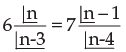or, 6 n (n – 1) (n – 2) = 7 (n – 1) (n – 2) (n – 3)
or, 6 n = 7 (n – 3)
or, 6 n = 7n – 21
or, n = 2 1
Therefore, the value of n equals 21.

Example 7 : Compute the sum of 4 digit numbers which can be formed with the four digits 1, 3, 5, 7, if each digit is used only once in each arrangement.
Solution : The number of arrangements of 4 different digits taken 4 at a time is given by 4P4 = 4! = 24. All the four digits will occur equal number of times at each of the position, namely ones, tens, hundreds, thousands.
Thus, each digit will occur 24 / 4 = 6 times in each of the position. The sum of digits in one’s position will be 6 × (1 + 3 + 5 + 7) = 96. Similar is the case in ten’s, hundred’s and thousand’s places. Therefore, the sum will be 96 + 96 × 10 + 96 × 100 + 96 × 1000 = 106656.

Example 8 : Find n if nP3 = 60.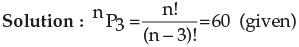i.e., n (n–1) (n–2) = 60 = 5 × 4 × 3
Therefore, n = 5.

Example 9 : If 56Pr+6 : 54Pr+3 = 30800 : 1, find r.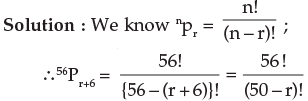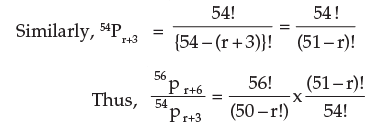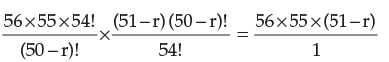But we are given the ratio as 30800 : 1 ; therefore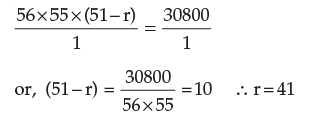Example 10 : Prove the following
(n + 1)! – n! = ⇒ n.n!

Solution : By applying the simple properties of factorial, we have
(n +1)! – n! = (n+1) n! – n! = n!. (n+1–1) = n. n!

Example 11 : In how many different ways can a club with 10 members select a President, Secretary and Treasurer, if no member can hold two offices and each member is eligible for any office?

Solution : The answer is the number of permutations of 10 persons chosen three at a time.
Therefore, it is 10p3 = 10×9×8=720.

Example 12 : When Jhon arrives in New York, he has eight shops to see, but he has time only to visit six of them. In how many different ways can he arrange his schedule in New York?

Solution : He can arrange his schedule in 8P6 = 8× 7 × 6 × 5 × 4 × 3 = 20160 ways.

Example 13 : When Dr. Ram arrives in his dispensary, he finds 12 patients waiting to see him.
If he can see only one patients at a time, find the number of ways, he can schedule his patients (a) if they all want their turn, and (b) if 3 leave in disgust before Dr. Ram gets around to seeing them.

Solution : (a) There are 12 patients and all 12 wait to see the doctor. Therefore the number of ways = 12P12 = 12! = 479,001,600

(b) There are 12–3 = 9 patients. They can be seen 12P9 = 79,833,600 ways.

CIRCULAR PERMUTATIONS

So for we have discussed arrangements of objects or things in a row which may be termed as linear permutation. But if we arrange the objects along a closed curve viz., a circle, the permutations are known as circular permutations.

The number of circular permutations of n different things chosen at a time is (n–1)!.
Proof : Let any one of the permutations of n different things taken. Then consider the rearrangement of this permutation by putting the last thing as the first thing. Eventhough this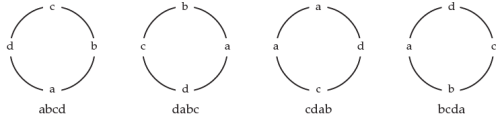is a different permutation in the ordinary sense, it will not be different in all n things are arranged in a circle. Similarly, we can consider shifting the last two things to the front and so on. Specially, it can be better understood, if we consider a,b,c,d. If we place a,b,c,d in order, then we also get abcd, dabc, cdab, bcda as four ordinary permutations. These four words in circular case are one and same thing. See above circles.

Thus we find in above illustration that four ordinary permutations equals  one in circular.
Therefore, n ordinary permutations equal one circular permutation.
Hence there are nPn/ n ways in which all the n things can be arranged in a circle. This equals (n–1)!.

Example 1 : In how many ways can 4 persons sit at a round table for a group discussions?

Solution : The answer can be get from the formula for circular permutations. The answer is (4–1)! = 3! = 6 ways.

NOTE :  These arrangements are such that every person has got the same two neighbours. The only change is that right side neighbour and vice-versa.
Thus the number of ways of arranging n persons along a round table so that no person has the same two neighbours is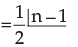Similarly, in forming a necklace or a garland there is no distinction between a clockwise and anti clockwise direction because we can simply turn it over so that clockwise becomes anti clockwise and vice versa. Hence, the number of necklaces formed with n beads of different coloursPERMUTATION WITH RESTRICTIONS

In many arrangements there may be number of restrictions. in such cases, we are to arrange or select the objects or persons as per the restrictions imposed. The total number of arrangements in all cases, can be found out by the application of fundamental principle.

Theorem 1. Number of permutations of n distinct objects when a particular object is not taken in any arrangement is n–1pr.

Proof : Since a particular object is always to be excluded, we have to place n – 1 objects at r places. Clearly this can be done in n–1pr ways.

Theorem 2. Number of permutations of n distinct objects when a particular object is always included in any arrangement is r. n–1pr–1.

Proof : If the particular object is placed at first place, remaining r – 1 places can be filled from n – 1 distinct objects in n–1pr–1 ways. Similarly, by placing the particular object in 2nd, 3rd, ….., rth place, we find that in each case the number of permutations is n–1pr–1.This the total number of arrangements in which a particular object always occurs is r. n–1pr–1 The following examples will enlighten further:

Example 1 : How many arrangements can be made out of the letters of the word DRAUGHT, the vowels never beings separated?

Solution : The word DRAUGHT consists of 7 letters of which 5 are consonants and two are vowels. In the arrangement we are to take all the 7 letters but the restriction is that the two vowels should not be separated.

We can view the two vowels as one letter. The two vowels A and U in this one letter can be arranged in 2! = 2 ways. (i) AU or (ii) UA. Further, we can arrange the six letters : 5 consonants and one letter compound letter consisting of two vowels. The total number of ways of arranging them is 6P = 6! = 720 ways.
Hence, by the fundamental principle, the total number of arrangements of the letters of the word DRAUGHT, the vowels never being separated = 2 × 720 = 1440 ways.

Example 2 : Show that the number of ways in which n books can be arranged on a shelf so that two particular books are not together.The number is (n–2).(n–1)!

Solution: We first find the total number of arrangements in which all n books can be arranged on the shelf without any restriction. The number is,nPn = n! ….. (1)
Then we find the total number of arrangements in which the two particular books are together.

The books can be together in 2P2 = 2! = 2 ways. Now we consider those two books which are kept together as one composite book and with the rest of the (n–2) books from (n–1) books which are to be arranged on the shelf; the number of arrangements = n–1Pn–1 = (n–1) !. Hence by the Fundamental Principle, the total number of arrangements on which the two particular books are together equals = 2 × (n–1)! …….(2)
the required number of arrangements of n books on a shelf so that two particular books are not together
= (1) – (2)
= n! – 2 x (n–1)!
= n.(n – 1)! – 2 . (n–1)!
=(n–1)! . (n–2)

Example 3 : There are 6 books on Economics, 3 on Mathematics and 2 on Accountancy. In how many ways can these be placed on a shelf if the books on the same subject are to be together?

Solution : Consider one such arrangement. 6 Economics books can be arranged among themselves in 6! Ways, 3 Mathematics books can be arranged in 3! Ways and the 2 books on Accountancy can be arranged in 2! ways. Consider the books on each subject as one unit. Now there are three units. These 3 units can be arranged in 3! Ways.
Total number of arrangements =  3! × 6! × 3! × 2!
=  51,840.

Example 4 : How many different numbers can be formed by using any three out of five digits 1, 2, 3, 4, 5, no digit being repeated in any number?
How many of these will (i) begin with a specified digit? (ii) begin with a specified digit and end with another specified digit?

Solution : Here we have 5 different digits and we have to find out the number of permutations of them 3 at a time. Required number is 5P3 = 5.4.3 = 60.
(i) If the numbers begin with a specified digit, then we have to find

The number of Permutations of the remaining 4 digits taken 2 at a time. Thus, desire number is 4P2 = 4.3 = 12.
(ii) Here two digits are fixed; first and last; hence, we are left with the choice of finding the number of permutations of 3 things taken one at a time i.e., 3P1 =3.

Example 5 : How many four digit numbers can be formed out of the digits 1,2,3,5,7,8,9, if no digit is repeated in any number? How many of these will be greater than 3000?

Solution : We are given 7 different digits and a four-digit number is to be formed using any 4 of these digits. This is same as the permutations of 7 different things taken 4 at a time.
Hence, the number of four-digit numbers that can be formed = 7P4 = 7 × 6 × 5 × 4 × = 840 ways.
Next, there is the restriction that the four-digit numbers so formed must be greater than 3,000. thus, it will be so if the first digit-that in the thousand’s position, is one of the five digits 3, 5, 7, 8, 9. Hence, the first digit can be chosen in 5 different ways; when this is done, the rest of the 3 digits are to be chosen from the rest of the 6 digits without any restriction and this can be done in 6P3 ways.
Hence, by the Fundamental principle, we have the number of four-digit numbers greater than 3,000 that can be formed by taking 4 digits from the given 7 digits = 5 × 6P= 5 × 6 × 5 × 4 = 5 × 120 = 600.

Example 6 : Find the total number of numbers greater than 2000 that can be formed with the digits 1, 2, 3, 4, 5 no digit being repeated in any number.

Solution : All the 5 digit numbers that can be formed with the given 5 digits are greater than 2000. This can be done in
5P5 = 5! = 120 ways …...................................(1)
The four digited numbers that can be formed with any four of the given 5 digits are greater than 2000 if the first digit, i.e.,the digit in the thousand’s position is one of the four digits 2, 3, 4, 5. this can be done in 4P1 = 4 ways. When this is done, the rest of the 3 digits are to be chosen from the rest of  5–1 = 4 digits. This can be done in 4P3 = 4 × 3 × 2 = 24 ways.
Therefore, by the Fundamental principle, the number of four-digit numbers greater than 2000 = 4 × 24 = 96 …. (2)
Adding (1) and (2), we find the total number greater than 2000 to be 120 + 96 = 216.

Example 7 : There are 6 students of whom 2 are Indians, 2 Americans, and the remaining 2 are Russians. They have to stand in a row for a photograph so that the two Indians are together, the two Americans are together and so also the two Russians. Find the number of ways in which they can do so.

Solution : The two Indians can stand together in 2P2 = 2! = 2 ways. So is the case with the two Americans and the two Russians.
Now these 3 groups of 2 each can stand in a row in 3P3 = 3 x 2 = 6 ways. Hence by the generalized fundamental principle, the total number of ways in which they can stand for a photograph under given conditions is 6 × 2 × 2 × 2 = 48

Example 8 : A family of 4 brothers and three sisters is to be arranged for a photograph in one row. In how many ways can they be seated if (i) all the sisters sit together, (ii) no two sisters sit together?

Solution : (i) Consider the sisters as one unit and each brother as one unit. 4 brother and 3 sisters make 5 units which can be arranged in 5! ways. Again 3 sisters may be arranged amongst themselves in 3! Ways Therefore, total number of ways in which all the sisters sit together = 5!×3! = 720 ways.
(ii) In this case, each sister must sit on each side of the brothers. There are 5 such positions as indicated below by upward arrows :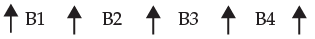4 brothers may be arranged among themselves in 4! ways. For each of these arrangements 3 sisters can sit in the 5 places in 5P3 ways.
Thus the total number of ways = 5P3 × 4! = 60 × 24 = 1,440

Example 9 : In how many ways can 8 persons be seated at a round table? In how many cases will 2 particular persons sit together?

Solution : This is in form of circular permutation. Hence the number of ways in which eight persons can be seated at a round table is (n – 1)! = (8 – 1)! = 7! = 5040 ways.
Consider the two particular persons as one person. Then the group of 8 persons becomes a group of 7 (with the restriction that the two particular persons be together) and seven persons can be arranged in a circular in 6! Ways.
Hence, by the fundamental principle, we have, the total number of cases in which 2 particular persons sit together in a circular arrangement of 8 persons = 2! × 6! = 2 × 6 × 5 × 4 × 3 ×2×1 = 1,440.

Example 10 : Six boys and five girls are to be seated for a photograph in a row such that no two girls sit together and no two boys sit together. Find the number of ways in which this can be done.

Solution : Suppose that we have 11 chairs in a row and we want the 6 boys and 5 girls to be seated such that no two girls and no two boys are together. If we number the chairs from left to right, the arrangement will be possible if and only if boys occupy the odd places and girls occupy the even places in the row. The six odd places from 1 to 11 may filled in by 6 boys in 6P6 ways. Similarly, the five even places from 2 to 10 may be filled in by 5 girls in 5P5 ways.
Hence, by the fundamental principle, the total number of required arrangements = 6P6 × 5P5 = 6! × 5! = 720 × 120 = 86400.

The document Permutation and Combinations (Part - 1) Notes | Study Business Mathematics and Logical Reasoning & Statistics - CA Foundation is a part of the CA Foundation Course Business Mathematics and Logical Reasoning & Statistics.
All you need of CA Foundation at this link: CA Foundation

## Business Mathematics and Logical Reasoning & Statistics

81 videos|101 docs|87 tests
 Use Code STAYHOME200 and get INR 200 additional OFF

## Business Mathematics and Logical Reasoning & Statistics

81 videos|101 docs|87 tests

Track your progress, build streaks, highlight & save important lessons and more!

,

,

,

,

,

,

,

,

,

,

,

,

,

,

,

,

,

,

,

,

,

;